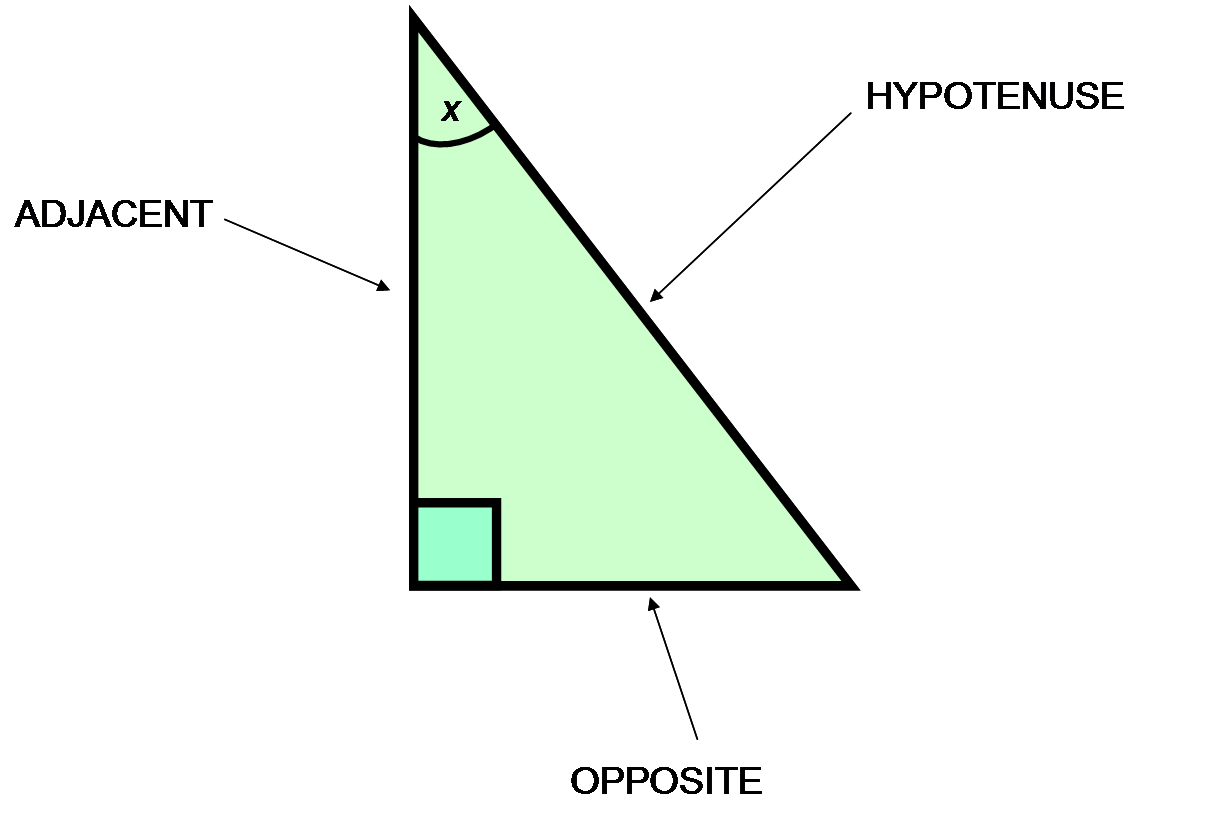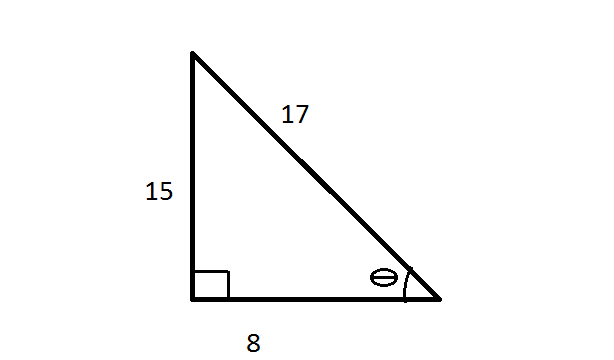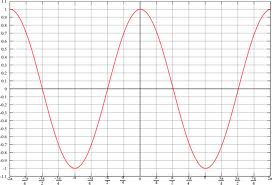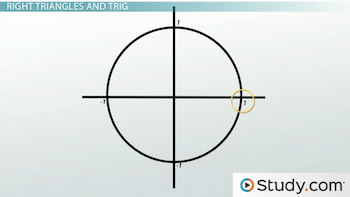# Cosine: Definition & Overview

Instructor: Jennifer Beddoe
The cosine function (cos) is one of the basic trigonometric functions. This lesson will define the cosine, explain how to use it to solve problems, and give some examples. The lesson ends with a quiz to help you retain what you have learned.

## Trigonometric Functions

There are six main trigonometric functions:

1. Sine (sin)
2. Cosine (cos)
3. Tangent (tan)
4. Secant (sec)
5. Cosecant (csc)
6. Cotangent (cot)

These functions are used to relate the angles of a triangle with the sides of that triangle. Trigonometric functions are important when studying triangles and modeling periodic phenomena, such as waves, sound, and light.

## The Cosine Function

The cosine function is defined as the ratio of the side of the triangle adjacent to the angle divided by the hypotenuse.One way to remember this ratio along with the ratios for the other most common trigonometric functions is with the mnemonic SOHCAHTOA:

SOH = Sine is Opposite over Hypotenuse

CAH = Cosine is Adjacent over Hypotenuse

TOA = Tangent is Opposite over Adjacent

This ratio can be used to solve problems involving distance or height, or if you need to know an angle measure.

Example:

What is cosΦ?Cosine is the ratio of the side adjacent to the angle over the hypotenuse.

cosΦ = 8/17

cosΦ = 0.4706

## The Cosine Function as a Periodic Function

Trigonometric functions are called periodic functions because they repeat over a given period.

Look at the graph of the cosine function:You can see that the graph repeats itself at a distance of 2π. Therefore, we can say that the cosine function has a period of 2π. Usually, when looking at the cosine function in this way, you don't use degree measure, but radians. Radian is the standard unit of angle measurement used in mathematics. A full circle is 2π radians, which is equal to 360°.

## Cosine and Its Relation to a Unit Circle

In trigonometry, a unit circle is a circle centered on the origin (0,0) of a coordinate plane with a radius of 1.Any point on the circle corresponds to the sine and cosine made by the angle created by a line from the center of the circle to that point and the x-axis. If the point is (x, y), the relationship looks like this -

sinθ = y

cosθ = x

For example:

Using the unit circle, determine the value of cos π/3?

To unlock this lesson you must be a Study.com Member.

### Register to view this lesson

Are you a student or a teacher?

### Unlock Your Education

#### See for yourself why 30 million people use Study.com

##### Become a Study.com member and start learning now.
Back
What teachers are saying about Study.com

### Earning College Credit

Did you know… We have over 160 college courses that prepare you to earn credit by exam that is accepted by over 1,500 colleges and universities. You can test out of the first two years of college and save thousands off your degree. Anyone can earn credit-by-exam regardless of age or education level.